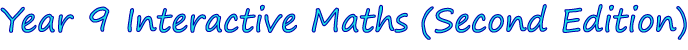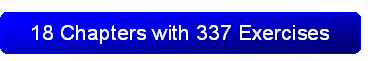1. The Distributive Law
2. Linear Equations and Inequalities
3. Pythagoras’ Theorem
4. Linear Graphs
5. Simultaneous Equations
6. Indices
7. Surds
8. Factors
9. Rational Expressions
11. Ratio and Proportion
12. Consumer Arithmetic
13. Geometry
14. Measurement
15. Trigonometry
16. Probability
17. Statistics
18. RevisionThe Year 9 Interactive Maths software is compatible with both Windows® and Mac® computers.  Discover more about Year 9 Interactive Maths by:

• Viewing the 17 chapter screen shots shown below that show step-by-step solutions in G S Rehill's proven writing style.  Students use the solutions after answering questions from the interactive exercises to identify mistakes in their working and to reinforce concepts, reasoning or mathematical laws.
• Clicking Mathematics Software Tutorials to watch 2 tutorials that show how students use the mathematics software.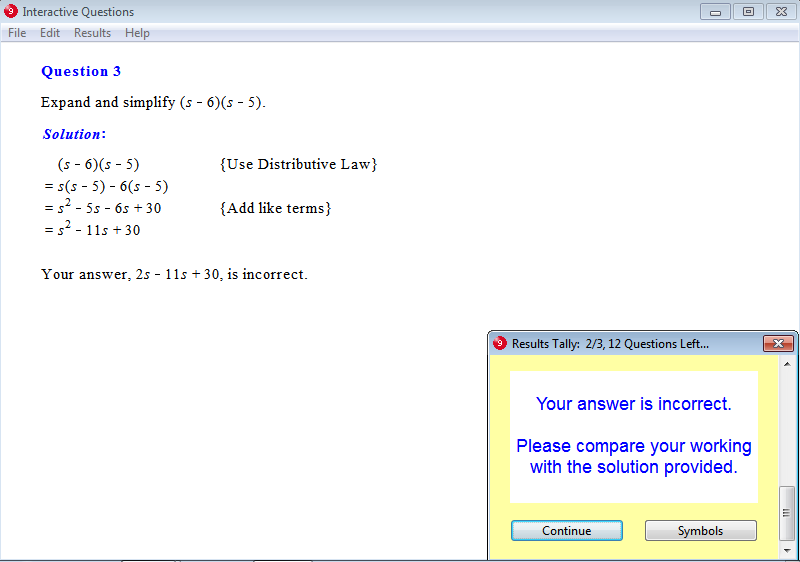From Chapter 1: The Distributive Law, Exercise 7: Binomial Expansions.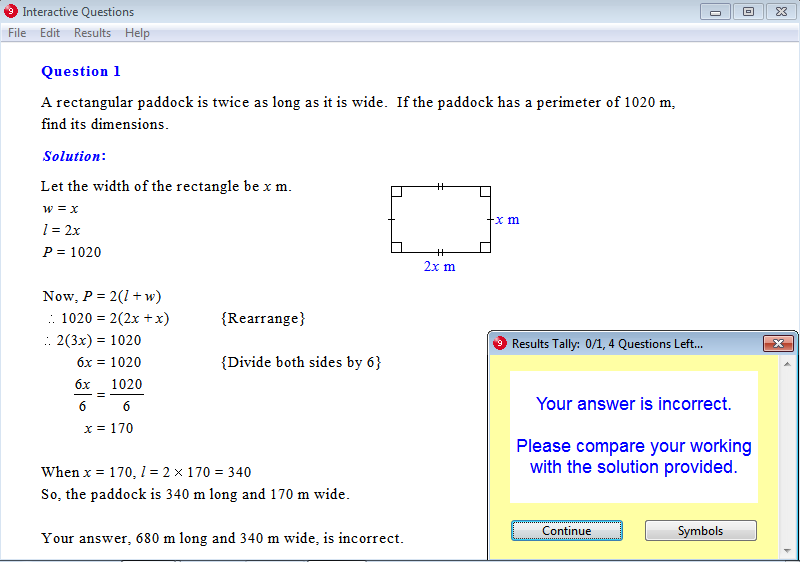From Chapter 2: Linear Equations and Inequalities, Exercise 16: Problem Solving.From Chapter 3: Pythagoras' Theorem, Exercise 15: Navigation Problems.From Chapter 4: Linear Graphs, Exercise 5: Sketch Graphs.From Chapter 5: Simultaneous Equations, Exercise 9: Problem Solving.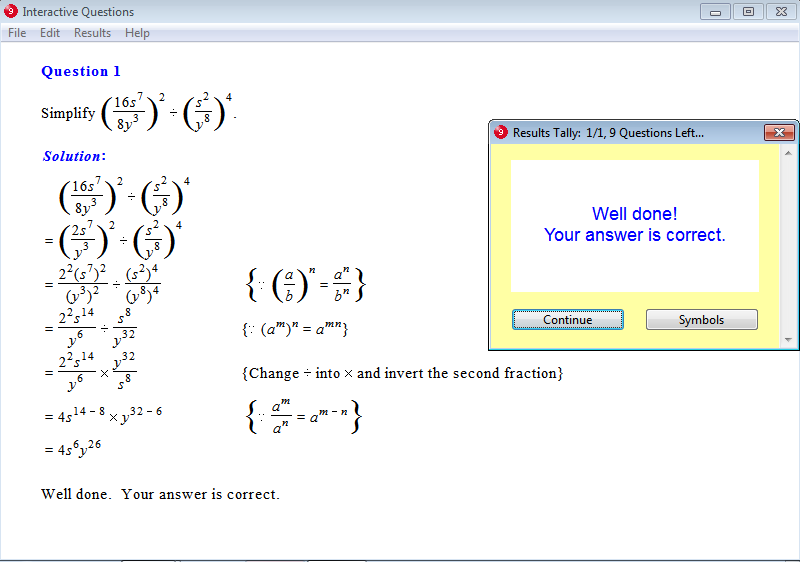From Chapter 6: Indices, Exercise 19: Index Law for Powers of Quotients.From Chapter 7: Surds, Exercise 11: Multiplication of Surds.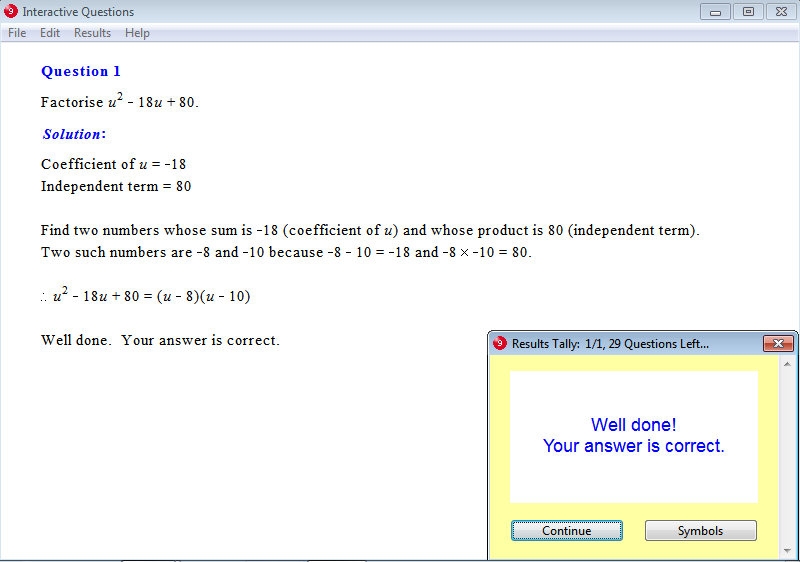From Chapter 8: Factors, Exercise 18: Factorisation of Quadratic Trinomials.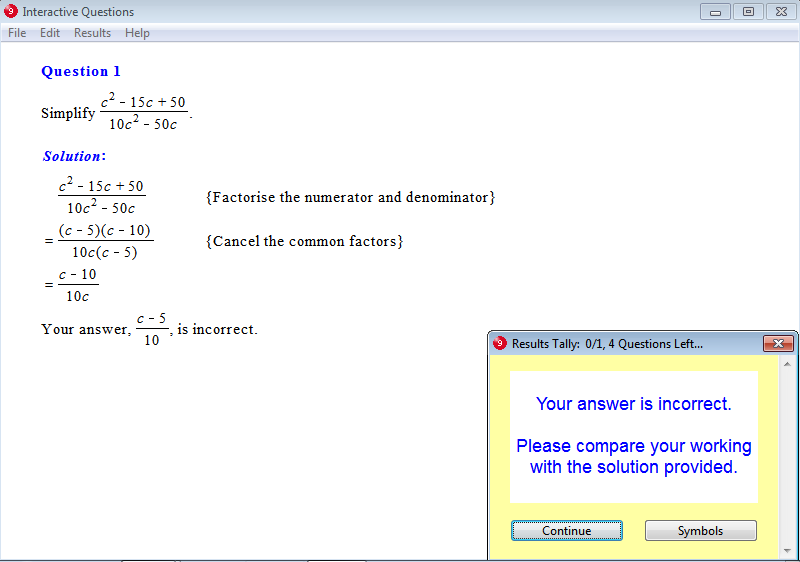From Chapter 9: Rational Expressions, Exercise 6: Simplification of Rational Expressions.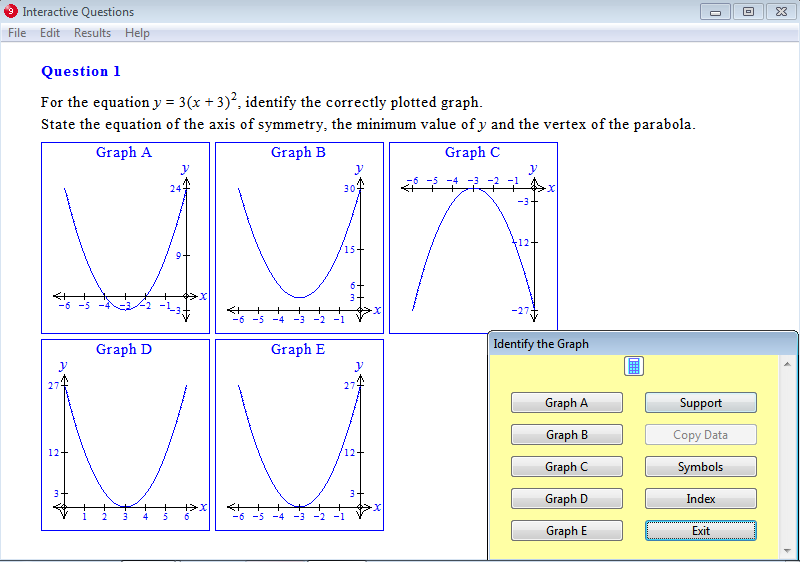From Chapter 10: Quadratic Equations and Graphs, Exercise 19: Graphs of y = a(x - b)², a > 0.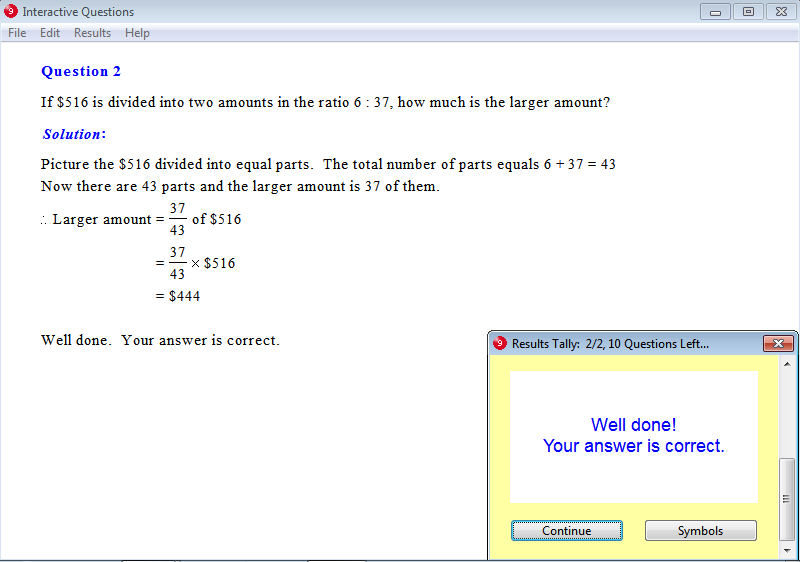From Chapter 11: Ratio and Proportion, Exercise 5: Dividing a Ratio in a Given Quantity.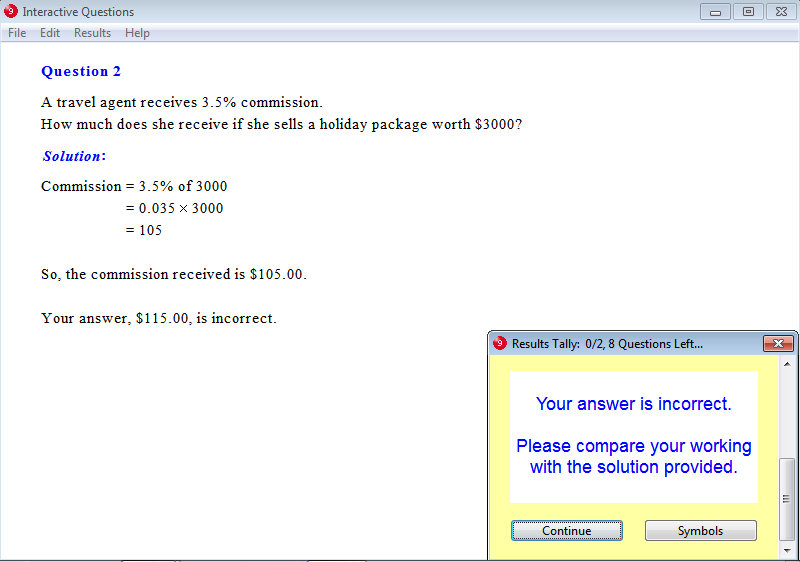From Chapter 12: Consumer Arithmetic, Exercise 21: Commission.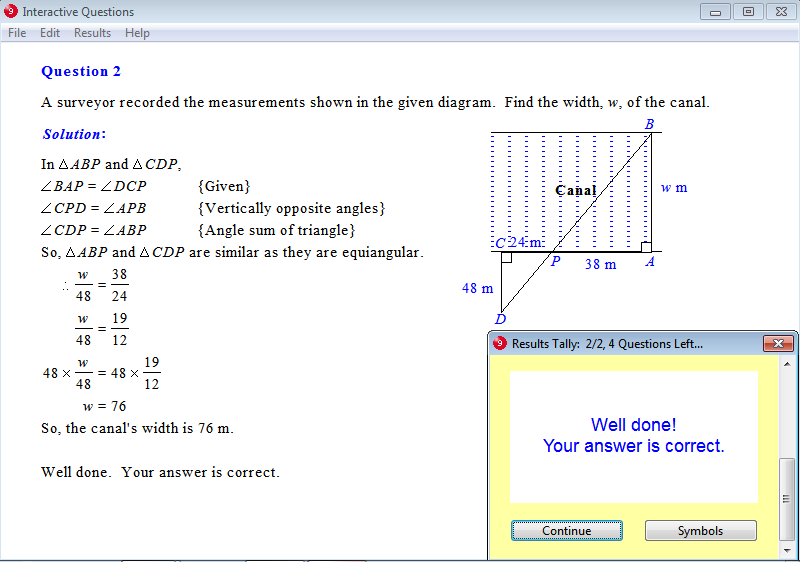From Chapter 13: Geometry, Exercise 24: Similar Triangles - Problem Solving.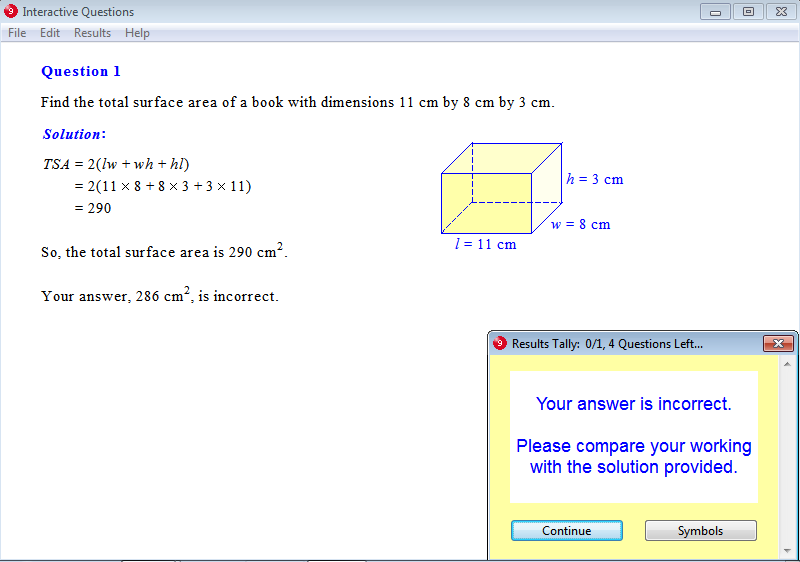From Chapter 14: Measurement, Exercise 16: Total Surface Area of a Cuboid.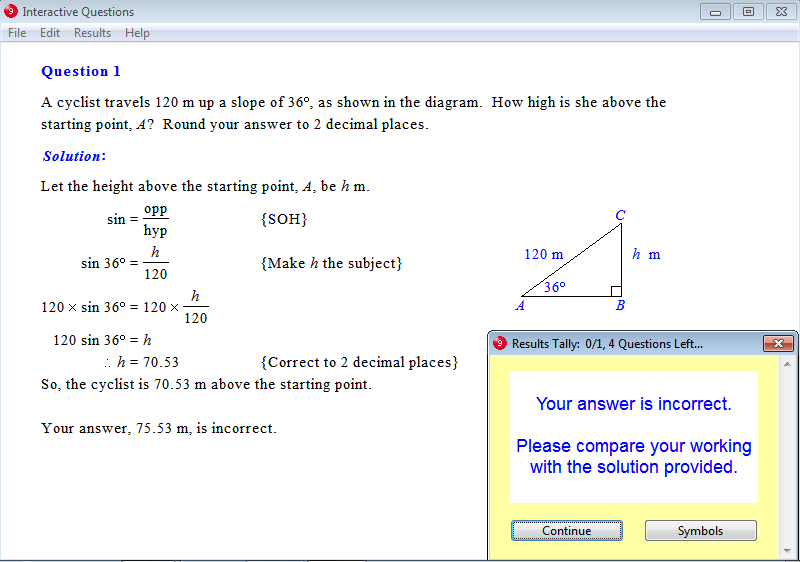From Chapter 15: Trigonometry, Exercise 17: Slope Problems.From Chapter 16: Probability, Exercise 4: Predictions.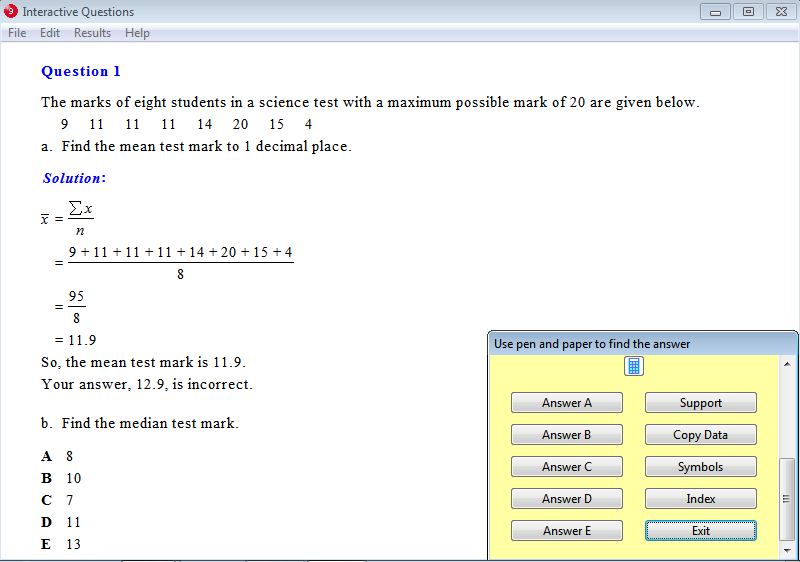From Chapter 17: Statistics, Exercise 1: Mean, Median and Mode.

Parents can order a 12-month Homework Licence for \$19.95 (AUD) per student by clicking this order button: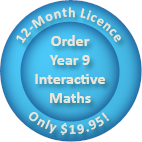Tutors may order Tutor Licences online that feature the interactive exercises as well as worksheets, tests and solution sheets.